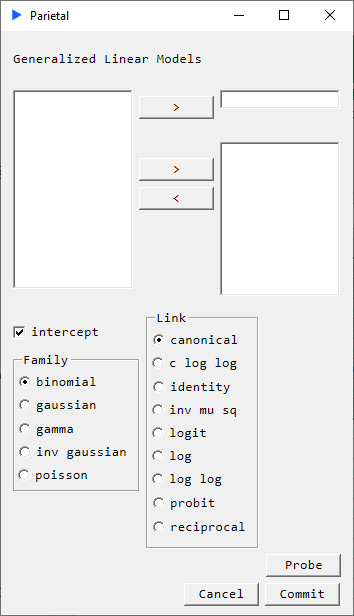# Generalized Linear Models

In cases where the dependent variable follows a distribution that is different from a normal distribution, we have a technique that generalizes OLS principles. This is known as the generalized linear model (GLM). If the target distribution falls under the *exponential family* of distributions, it uses a link function as a bridge to map the regression. Typically the method of iteratively reweighted least squares is used to fit the model due to quick convergence properties.

- Families
- Binomial
- Gaussian
- Gamma
- Inverse Gaussian
- Poisson
- Canonical
- C Log Log
- Identity
- Inverse MuSq
- Logit
- Log
- Log Log
- Probit
- Reciprocal##### Description#

For a GLM, three pieces are needed:

• A distribution belonging to the exponential family
• A linear predictor
• A link function that maps the linear predictor to the target distribution

The exponential family of distributions are ones whose density functions can be expressed in the form of:

$$f_Y(\mathbf{y} \mid \boldsymbol\theta, \tau) = h(\mathbf{y},\tau) \exp \left(\frac{\mathbf{b}(\boldsymbol\theta)^{\rm T}\mathbf{T}(\mathbf{y}) - A(\boldsymbol\theta)} {d(\tau)} \right)$$

We implement the following families:

• Binomial
• Gaussian
• Gamma
• Inverse Gaussian
• Poisson

We describe the linear predictor as. $$\eta = X \beta$$

Then we define a link function that maps this linear predictor to mean of the target distribution. $$E(Y \mid X) = \mu = g^{-1}(\eta)$$

We implement the following links:

Canonical (will choose the default link for the chosen Family)
Family: Gaussian     → Link: Identity
Family: Binomial     → Link: Logit
Family: Poisson      → Link: Log
Family: Gamma        → Link: Reciprocal
Family: Inv Gaussian → Link: Inverse Mu Squared
C Log Log
Identity
Inverse Mu Squared
Logit
Log
LogLog
Probit
Reciprocal

##### Returns#
• coefficient
• standard error
• t-statisitc
• p-value
• convergence
• fisher iterations
• residual null
• residual deviance